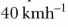# What is the difference between uniform velocity and non-uniform velocity?

What is the difference between uniform velocity and non-uniform velocity?

Uniform velocity: An object with uniform velocity covers equal distances in equal intervals of time in a specified direction, e.g., an object moving with speed oftowards west has uniform velocity. Non-uniform velocity: When an object covers unequal distances in equal intervals of time in a specified direction, or if the direction of motion changes, it is said to be moving with a non-uniform or variable velocity, e.g., revolving fan at a constant speed has variable velocity.